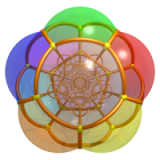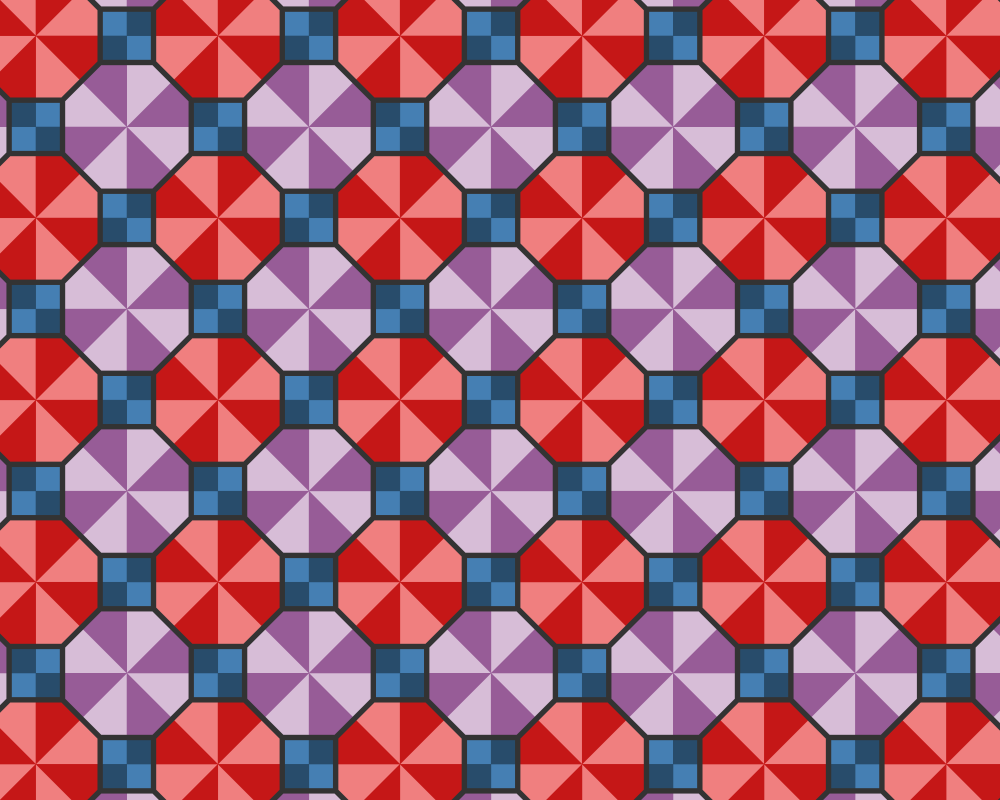# 静电场与 Marden 定理

1. 在复平面上不共线的三个点 $A,B,C$ 处各自放置一个带有单位正电荷，则电场强度为 0 的点都位于 $\Delta ABC$ 的内部，这样的点有两个，它们是三次复多项式 $P(z) = (z-A)(z-B)(z-C)$ 的导数 $P'(z)$ 的零点。
2. 不仅如此，这两个零点还是一个内切于 $\Delta ABC$ 的椭圆的焦点，此椭圆是所有内切于 $\Delta ABC$ 的椭圆中面积最大者，并且其与 $\Delta ABC$ 的三边的切点均为各边中点。这个椭圆叫做 Steiner 内切椭圆

# 具有超理想顶点的双曲蜂巢# 例子

• 下图是二维的欧式密铺 omnitruncated (4, 2, 4)：# Todd-Coxeter 算法和 3D/4D 均匀多胞体

The English version of this doc is here.

# Möbius 变换的分类与上半双曲空间的等距

2021/06/27 更新：我更新了一下 shader 代码，把每个动画放在一个 SVG 图片中。最后几个动画代码是可以使用键盘选择场景的，具体操作如下：

• 按下 1 开启/关闭 Möbius 变换。
• 按下 2 开启/关闭椭圆旋转。
• 按下 3 开启/关闭双曲缩放。
• 按下 4 开启/关闭展示 Riemann 球面。

1. Möbius 变换作为扩充复平面 $\hat{\mathbb{C}}$ 到自身的全纯函数。
2. Möbius 变换作为 Riemann 球面 $S^2$ 到自身的全纯函数。
3. Möbius 变换作为上半双曲空间中的等距变换。

1. 维基百科页面.
2. Visual complex analysis, Tristan Needham.

|